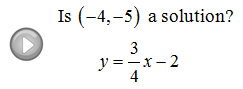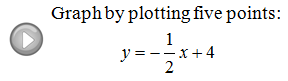## Algebra

### Graph by Plotting Points

Graphing lines can be done in a number of ways.  This section describes a method, plotting points, that always works and can be used for many other types of equations. Notice that in a linear equation with two variables, y = 3x - 2, the y-value depends on what the x-value is. Since x is independent here, choose any real number, say x = 4, and you can find the corresponding y-value by evaluating y = 3(4) – 2 = 12 – 2 = 10. Therefore, the ordered pair (4, 10) is a point on the graph of the equation.

Example: Graph y = −2x + 6 by plotting five points.

Step 1: Choose any five x-values.
Step 2: Evaluate to find the corresponding y-values.
Step 3: Plot the points and use a straight edge to draw a line through them.
When choosing x-values it is wise to pick some negative numbers as well as zero.  Try to find the points where the line crosses the x and y axes.  These special points are called the x- and y-intercepts.

Is the given point a solution?
Remember that we are less likely to make a mistake if we insert parentheses where we see a variable and then substitute in the appropriate values.

Find the corresponding value.
Graph by plotting points.

Example: Graph y = 2x − 4 by plotting five points.
Example: Graph y = −− 2 by plotting five points.
Choosing a scale when creating a blank coordinate system will take some thought.  Keep in mind that the scale on the x-axis need not be the same as the scale on the y-axis.

Example: Graph y = 1/2 x − 6 by plotting five points.
When the coefficient of x is a fraction, choose x-values to be multiples of the denominator so that you might avoid unnecessarily tedious calculations.

Example: Graph y = −3/2 x + 6 by plotting five points.
Example: Graph 2− 3y = 6 by plotting five points.
When dividing a binomial by a number you must divide both terms by that number.  For example, treat the −3 as a common denominator as in the previous problem.
A common error is to just divide the 6 by −3.